# Alternate Interior Angles Theorem

Alternate Interior Angles Theorem

If you hold a stick across the horizontal parallel bars of a window as depicted in the figure. How many angles will be formed between the horizontal bars and the stick?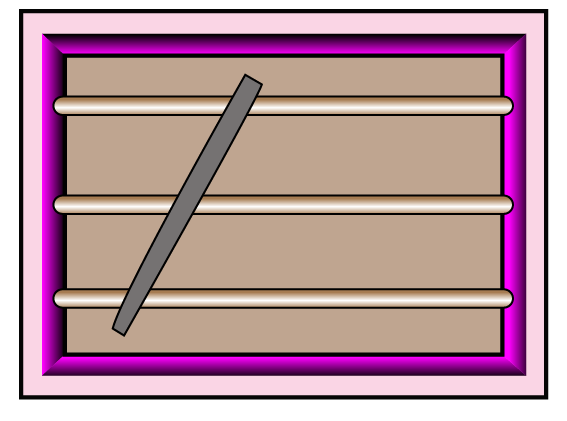A lot of special angle-pairs are formed, which are congruent. Alternate interior angles are one such congruent pair.

## Lesson Plan

 1 What Does the Alternate Interior Angles Theorem States? 2 Challenging Questions on Alternate Interior Angles Theorem 3 Important Notes on Alternate Interior Angles Theorem 4 Solved Examples on Alternate Interior Angles Theorem 5 Interactive Questions on Alternate Interior Angles Theorem

## What Are Parallel Lines and Transversal line?

Two lines that never intersect, are equidistant, and are coplanar are called parallel lines. The symbol for "parallel to" is II. Here line $$m$$ II $$n$$.

If we have two lines (they don't have to be parallel) and have a third line that crosses them, then the crossing line is said to be a transversal.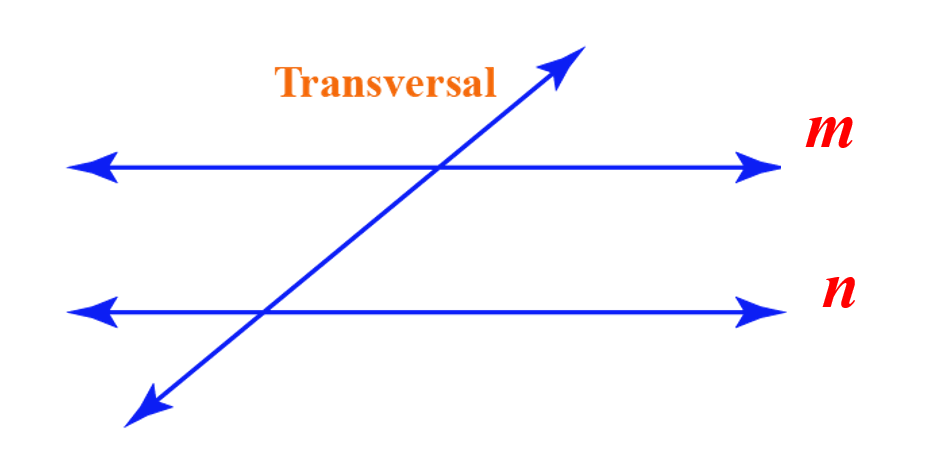<<create a similar image>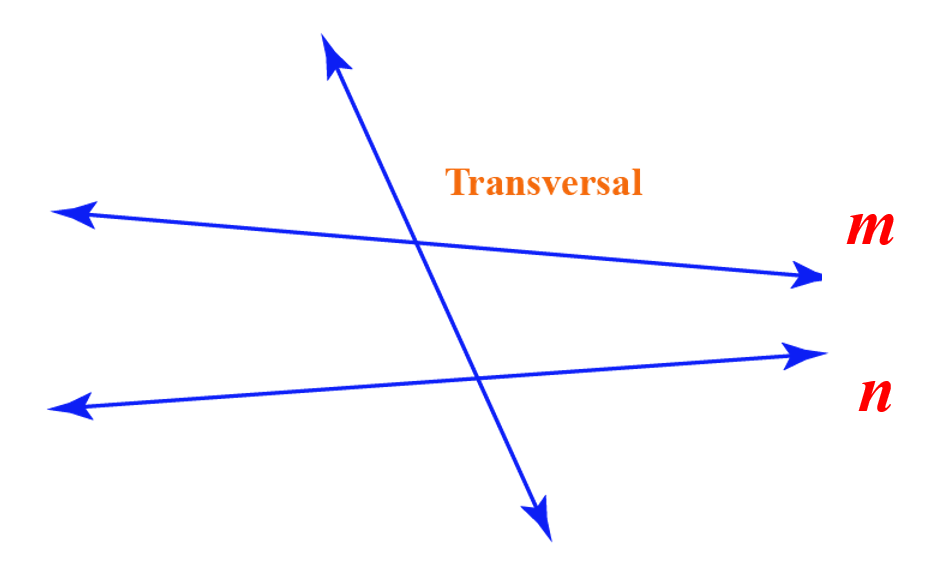<Create a similar image, name

## What Are Alternate Interior Angles?

Alternate angles are of two types:

1. Alternate interior angles
2. Alternate exterior angles

Alternate interior angles: Alternate interior angles are the angles formed when a transversal intersects two coplanar lines. They are on the inner side of the coplanar lines but are on the alternate opposite sides of the transversal.

Here, in the image shown below,

Alternate interior angles are:

1. $$\angle 3$$ and $$\angle 6$$

2. $$\angle 4$$ and $$\angle 5$$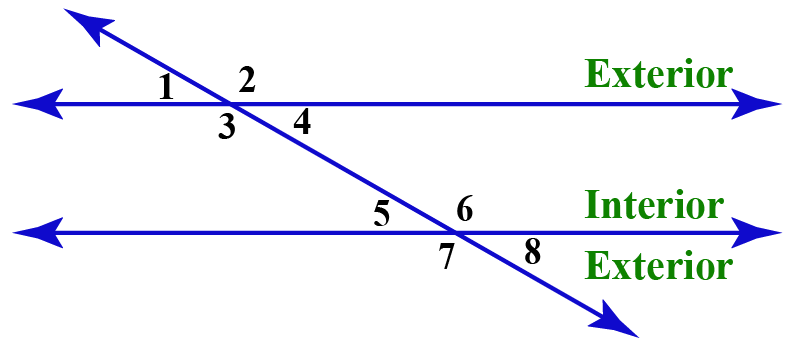Alternate exterior angles: Alternate exterior angles are formed on the exterior of the coplanar lines but on the alternate opposite sides of the transversal.

Here, in the image shown above,

Alternate exterior angles are:

1. $$\angle 1$$ and $$\angle 8$$

2. $$\angle 2$$ and $$\angle 7$$

## Alternate Interior Angles Examples

Let us try to spot alternate interior angles in the given figure. Remember the lines do not have to be always parallel for alternate angles to be formed.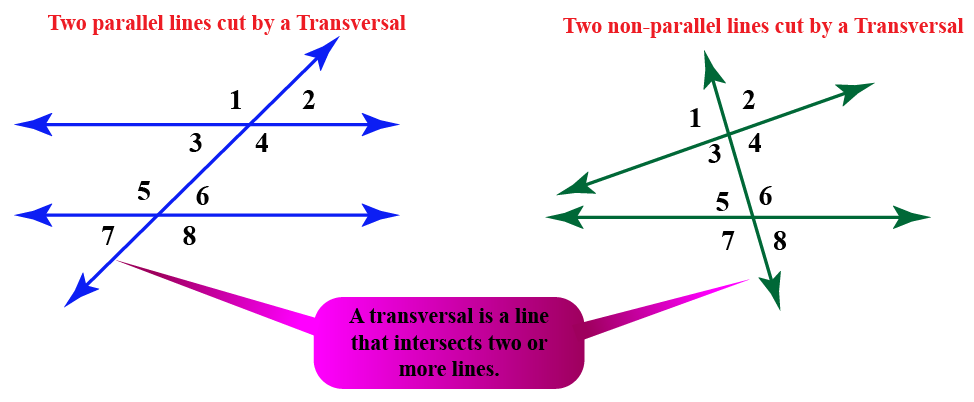Alternate interior angles are:

1. $$\angle 3$$ and $$\angle 6$$

2. $$\angle 4$$ and $$\angle 5$$

Alternate exterior angles are:

1. $$\angle 1$$ and $$\angle 8$$

2. $$\angle 2$$ and $$\angle 7$$

## How Do You Find Alternate Interior Angles?

Z-test For Finding Alternate Interior Angles
To find the alternate interior angles, make a zig-zag line including the parallel lines as shown in the diagram. Here, $$\text p$$ and $$\text q$$ are alternate interior angles. Similarly, $$\text a$$ and $$\text d$$ are also alternate interior angles.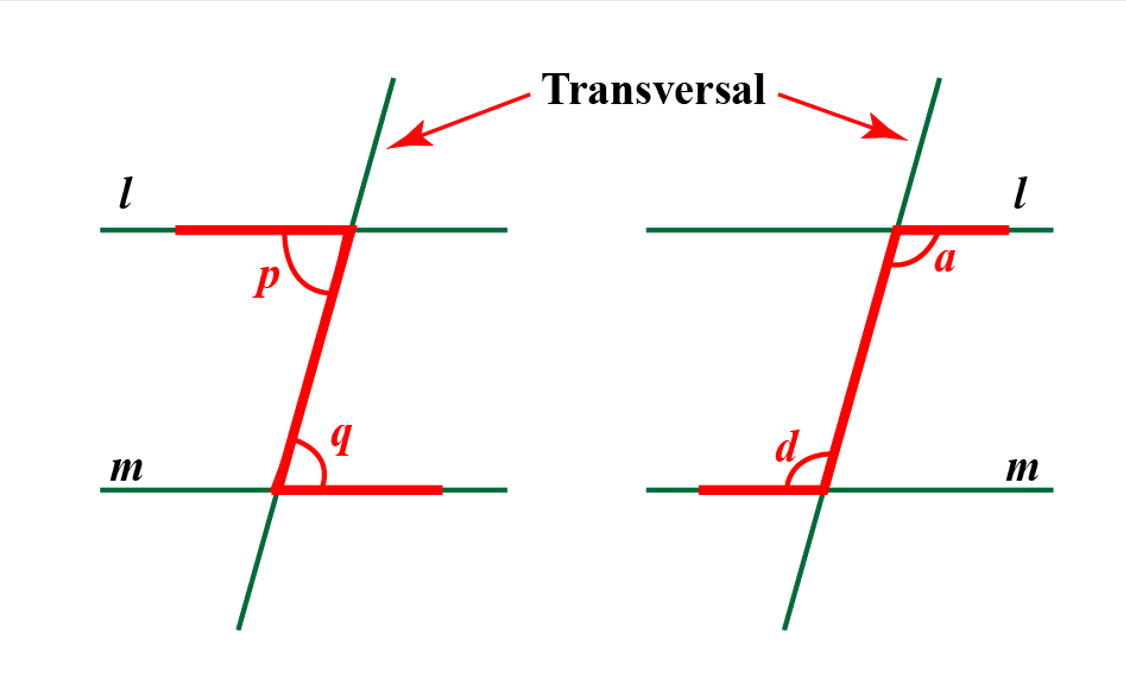## What Does the Alternate Interior Angles Theorem States?

The alternate interior angles theorem states that “if a transversal crosses the set of parallel lines, the alternate interior angles are congruent.”

In the figure given below, a set of parallel lines $$m$$ and $$n$$ are intersected by the transversal and the following pairs of alternate interior angles are formed:

$$\angle 1$$ and $$\angle 2$$

$$\angle 3$$ and $$\angle 4$$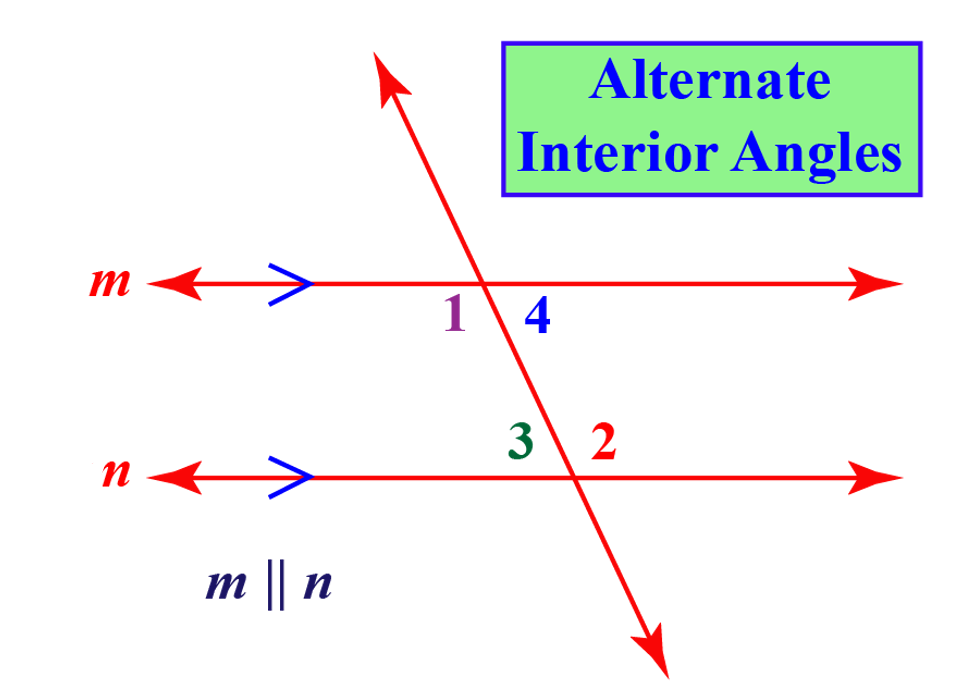Since the given lines $$m$$ and $$n$$ are parallel, therefore the alternate interior angles will be congruent.

$\angle 1 = \angle 2$

$\angle 3 = \angle 4$

• Interior angles on the same side of the transversal are called 'consecutive interior angles' or co-interior angles in short. Co-interior angles are supplementary when the lines are parallel.

$\angle 2 + \angle 3 = 180^{\circ}$

$\angle 6 + \angle 7 = 180^{\circ}$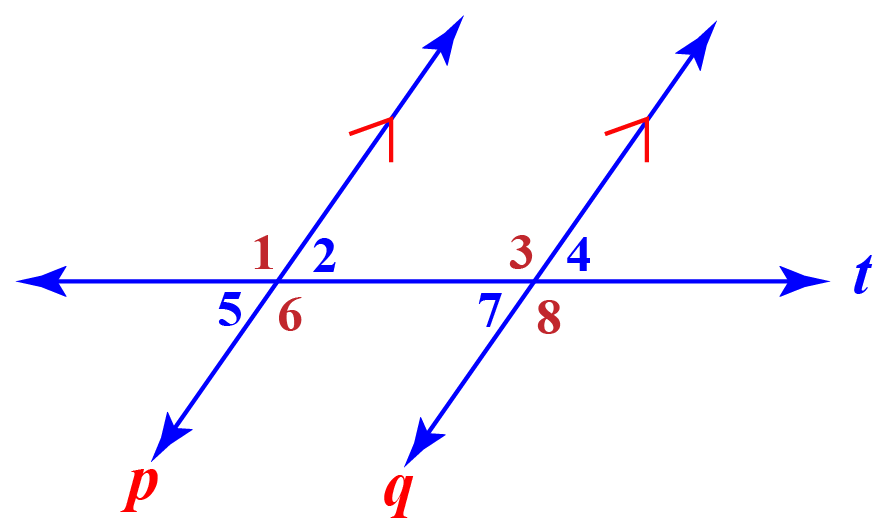• Corresponding angles are a pair of angles on the similar corners of each of two lines on the same side of the transversal line. Corresponding angles formed by two parallel lines and a transversal are equal.

Corresponding angles are:

1. $$\angle 2$$ and $$\angle 4$$

2. $$\angle 1$$ and $$\angle 3$$

3. $$\angle 5$$ and $$\angle 7$$

4. $$\angle 6$$ and $$\angle 8$$

## Explore Alternate Interior Angles Congruence

You can explore the alternate interior angle congruence in this simulation. Drag the red point to change the angle between parallel lines and the transversal.Challenging Question
• Find the value of $$x$$, if two congruent alternate interior angles are $$(2 x + 19)^{\circ}$$ and $$(4x -16)^{\circ}$$.

## What Is the Alternate Interior Angles Theorem?

### Theorem And Proof

Statement: The theorem states that  “if a transversal intersects parallel lines, the alternate interior angles are congruent."

Given: Line p II line q

To prove: $$\angle 2 = \angle 7$$ and $$\angle 3 = \angle 6$$Proof: Suppose $$p$$ and $$q$$ are two parallel lines and t is the transversal that intersects $$p$$ and $$q$$.

We know that, if a transversal intersects any two parallel lines, the corresponding angles and vertically opposite angles are congruent.

Therefore,

$$\angle 1 = \angle 3$$ ………..(I)  [Corresponding angles]

$$\angle 1 = \angle 6$$ ………..(II) [Vertically opposite angles]

From equations (I) and (II), we get-

$$\angle 3 = \angle 6$$ ..................[Alternate interior angles]

Similarly,

$$\angle 2 = \angle 7$$

Hence, it is proved.

## Alternate Interior Angles Theorem Converse

The converse of alternate interior angles theorem states that if two lines are intersected by a transversal forming congruent alternate interior angles, then the lines are parallel.

Thus according to the converse of alternate interior angles theorem, the below-given lines will be parallel if $$\angle D$$ is $$40^{\circ}$$ and $$\angle B$$ is $$140^{\circ}$$. This is because their corresponding alternate interior angles are of the measure $$40^{\circ}$$ and $$140^{\circ}$$.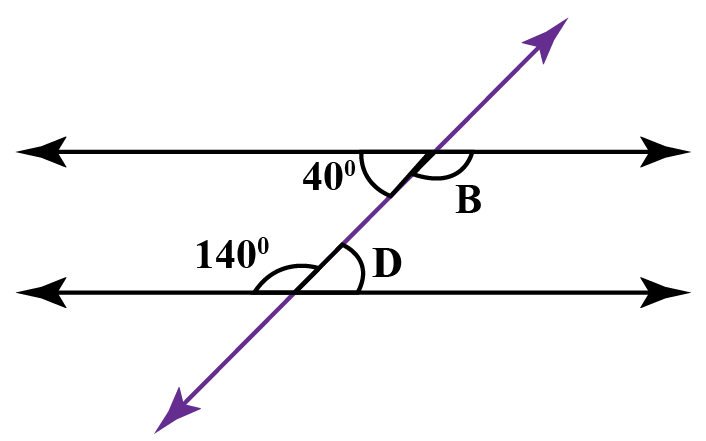In conclusion, the alternate interior angles theorem states that the alternate interior angles will be equal if the lines are parallel, whereas its converse states that lines will be parallel if the alternate interior angles are congruent.

More Important Topics
Numbers
Algebra
Geometry
Measurement
Money
Data
Trigonometry
Calculus